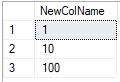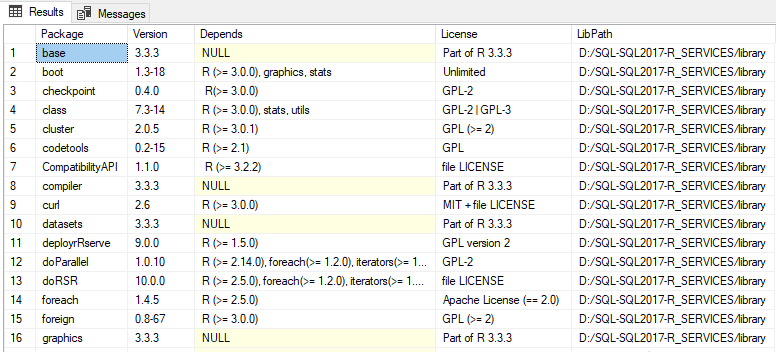# Quickstart: Run simple R scripts with SQL machine learning

Applies to:SQL Server 2016 (13.x) and laterAzure SQL Managed Instance

In this quickstart, you'll run a set of simple R scripts using SQL Server Machine Learning Services or on Big Data Clusters. You'll learn how to use the stored procedure sp_execute_external_script to execute the script in a SQL Server instance.

In this quickstart, you'll run a set of simple R scripts using SQL Server Machine Learning Services. You'll learn how to use the stored procedure sp_execute_external_script to execute the script in a SQL Server instance.

In this quickstart, you'll run a set of simple R scripts using SQL Server R Services. You'll learn how to use the stored procedure sp_execute_external_script to execute the script in a SQL Server instance.

In this quickstart, you'll run a set of simple R scripts using Azure SQL Managed Instance Machine Learning Services. You'll learn how to use the stored procedure sp_execute_external_script to execute the script in your database.

## Prerequisites

You need the following prerequisites to run this quickstart.

• A tool for running SQL queries that contain R scripts. This quickstart uses Azure Data Studio.

## Run a simple script

To run an R script, you'll pass it as an argument to the system stored procedure, sp_execute_external_script. This system stored procedure starts the R runtime, passes data to R, manages R user sessions securely, and returns any results to the client.

In the following steps, you'll run this example R script:

``````a <- 1
b <- 2
c <- a/b
d <- a*b
print(c(c, d))
``````
1. Open Azure Data Studio and connect to your server.

2. Pass the complete R script to the `sp_execute_external_script` stored procedure.

The script is passed through the `@script` argument. Everything inside the `@script` argument must be valid R code.

``````EXECUTE sp_execute_external_script @language = N'R'
, @script = N'
a <- 1
b <- 2
c <- a/b
d <- a*b
print(c(c, d))
'
``````
3. The correct result is calculated and the R `print` function returns the result to the Messages window.

It should look something like this.

Results

``````STDOUT message(s) from external script:
0.5 2
``````

## Run a Hello World script

A typical example script is one that just outputs the string "Hello World". Run the following command.

``````EXECUTE sp_execute_external_script @language = N'R'
, @script = N'OutputDataSet<-InputDataSet'
, @input_data_1 = N'SELECT 1 AS hello'
WITH RESULT SETS(([Hello World] INT));
GO
``````

Inputs to the `sp_execute_external_script` stored procedure include:

Input Description
@language defines the language extension to call, in this case, R
@script defines the commands passed to the R runtime. Your entire R script must be enclosed in this argument, as Unicode text. You could also add the text to a variable of type nvarchar and then call the variable
@input_data_1 data returned by the query, passed to the R runtime, which returns the data as a data frame
WITH RESULT SETS clause defines the schema of the returned data table, adding "Hello World" as the column name, int for the data type

The command outputs the following text:

Hello World
1

## Use inputs and outputs

By default, `sp_execute_external_script` accepts a single dataset as input, which typically you supply in the form of a valid SQL query. It then returns a single R data frame as output.

For now, let's use the default input and output variables of `sp_execute_external_script`: InputDataSet and OutputDataSet.

1. Create a small table of test data.

``````CREATE TABLE RTestData (col1 INT NOT NULL)

INSERT INTO RTestData
VALUES (1);

INSERT INTO RTestData
VALUES (10);

INSERT INTO RTestData
VALUES (100);
GO
``````
2. Use the `SELECT` statement to query the table.

``````SELECT *
FROM RTestData
``````

Results3. Run the following R script. It retrieves the data from the table using the `SELECT` statement, passes it through the R runtime, and returns the data as a data frame. The `WITH RESULT SETS` clause defines the schema of the returned data table for SQL, adding the column name NewColName.

``````EXECUTE sp_execute_external_script @language = N'R'
, @script = N'OutputDataSet <- InputDataSet;'
, @input_data_1 = N'SELECT * FROM RTestData;'
WITH RESULT SETS(([NewColName] INT NOT NULL));
``````

Results4. Now let's change the names of the input and output variables. The default input and output variable names are InputDataSet and OutputDataSet, this script changes the names to SQL_in and SQL_out:

``````EXECUTE sp_execute_external_script @language = N'R'
, @script = N' SQL_out <- SQL_in;'
, @input_data_1 = N' SELECT 12 as Col;'
, @input_data_1_name = N'SQL_in'
, @output_data_1_name = N'SQL_out'
WITH RESULT SETS(([NewColName] INT NOT NULL));
``````

Note that R is case-sensitive. The input and output variables used in the R script (SQL_out, SQL_in) need to match the names defined with `@input_data_1_name` and `@output_data_1_name`, including case.

Tip

Only one input dataset can be passed as a parameter, and you can return only one dataset. However, you can call other datasets from inside your R code and you can return outputs of other types in addition to the dataset. You can also add the OUTPUT keyword to any parameter to have it returned with the results.

5. You also can generate values just using the R script with no input data (`@input_data_1` is set to blank).

The following script outputs the text "hello" and "world".

``````EXECUTE sp_execute_external_script @language = N'R'
, @script = N'
mytextvariable <- c("hello", " ", "world");
OutputDataSet <- as.data.frame(mytextvariable);
'
, @input_data_1 = N''
WITH RESULT SETS(([Col1] CHAR(20) NOT NULL));
``````

Results@script as input" />

## Check R version

If you would like to see which version of R is installed, run the following script.

``````EXECUTE sp_execute_external_script @language = N'R'
, @script = N'print(version)';
GO
``````

The R `print` function returns the version to the Messages window. In the example output below, you can see that in this case, R version 3.4.4 is installed.

Results

``````STDOUT message(s) from external script:
_
platform       x86_64-w64-mingw32
arch           x86_64
os             mingw32
system         x86_64, mingw32
status
major          3
minor          4.4
year           2018
month          03
day            15
svn rev        74408
language       R
version.string R version 3.4.4 (2018-03-15)
nickname       Someone to Lean On
``````

## List R packages

Microsoft provides a number of R packages pre-installed with Machine Learning Services.

Microsoft provides a number of R packages pre-installed with R Services.

To see a list of which R packages are installed, including version, dependencies, license, and library path information, run the following script.

``````EXEC sp_execute_external_script @language = N'R'
, @script = N'
OutputDataSet <- data.frame(installed.packages()[,c("Package", "Version", "Depends", "License", "LibPath")]);'
WITH result sets((
Package NVARCHAR(255)
, Version NVARCHAR(100)
, Depends NVARCHAR(4000)
The output is from `installed.packages()` in R and is returned as a result set.